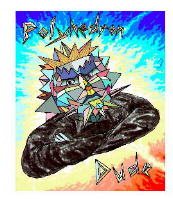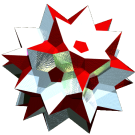Acronym presipsido Name pseudoretrosnub pentagonal snub (pseudo)disoctahedron,compound of 2 gad `© ©` Circumradius sqrt[(5+sqrt(5))/8] = 0.951057 Inradius sqrt[(5+sqrt(5))/40] = 0.425325 Vertex figure /2 General of army x3f4o Colonel of regiment siddo Dihedral angles (at margins) between {5} and {5}:   arccos(1/sqrt(5)) = 63.434949° ExternallinksIncidence matrix

```  24 |  5 |  5 || 1
-----+----+----++--
2 | 60 |  2 || 1
-----+----+----++--
5 |  5 | 24 || 1
-----+----+----++--
♦ 12 | 30 | 12 || 2
```